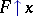# Transfinite recursion

(diff) ← Older revision | Latest revision (diff) | Newer revision → (diff)(*)
whereis the "piece" of the functionto be defined restricted to the set of predecessors of, andis some given function. Many functions and classes important in set theory (for example, the class of Gödel constructible sets, cf. Gödel constructive set) are defined by means of transfinite recursion. The analogy between this set-theoretic construction and the recursive definitions of numerical functions has been an object of study in the theory of algorithms from the very beginning of its creation (cf. Algorithms, theory of). In particular, this analogy lies at the basis of the various classifications of general recursive functions (see ).
Of great significance in a number of branches of mathematical logic (proof theory, recursive hierarchy theory) are the recursive ordinal numbers and the modification of transfinite recursion related to them. (Recursive ordinal numbers are the algorithmic analogues of countable ordinal numbers; subsequently, analogues of ordinal numbers of larger cardinality were proposed.) In this situation, transfinite recursion is called the Rogers lemma. Here, equation (*) is subjected to the following modification: The functionis replaced by an arbitrary recursive function, and instead of the function, its Gödel number is used (i.e. the code of its algorithmic description, see also Arithmetization).